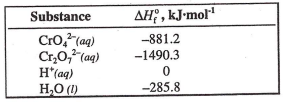# Problem: Use the standard enthalpies of formation in the table to calculate Δ H° for this reaction. 2 CrO42- (aq) + 2 H+ (aq) → Cr2O72- (aq) + H2O (l) a) +272.1 kJ b) +13.7 kJ c) -13.7 kJ d) -272.1 kJ

###### FREE Expert Solution
99% (203 ratings)
###### Problem Details

Use the standard enthalpies of formation in the table to calculate Δ H° for this reaction.

2 CrO42- (aq) + 2 H(aq) → Cr2O72- (aq) + H2O (l)

a) +272.1 kJ

b) +13.7 kJ

c) -13.7 kJ

d) -272.1 kJWhat scientific concept do you need to know in order to solve this problem?

Our tutors have indicated that to solve this problem you will need to apply the Enthalpy of Formation concept. You can view video lessons to learn Enthalpy of Formation. Or if you need more Enthalpy of Formation practice, you can also practice Enthalpy of Formation practice problems.

What is the difficulty of this problem?

Our tutors rated the difficulty ofUse the standard enthalpies of formation in the table to cal...as medium difficulty.

How long does this problem take to solve?

Our expert Chemistry tutor, Sabrina took 4 minutes and 22 seconds to solve this problem. You can follow their steps in the video explanation above.

What professor is this problem relevant for?

Based on our data, we think this problem is relevant for Professor Brynteson's class at LU.